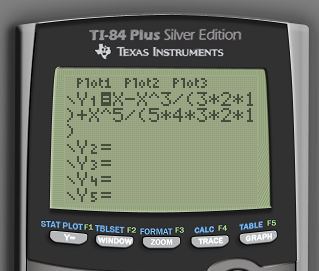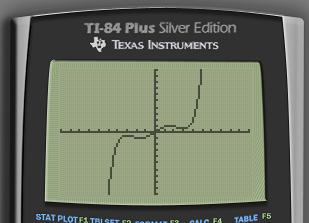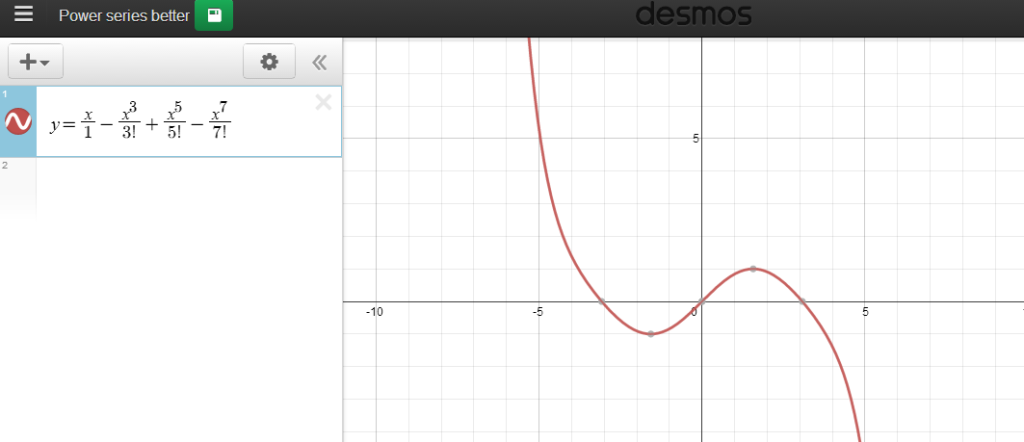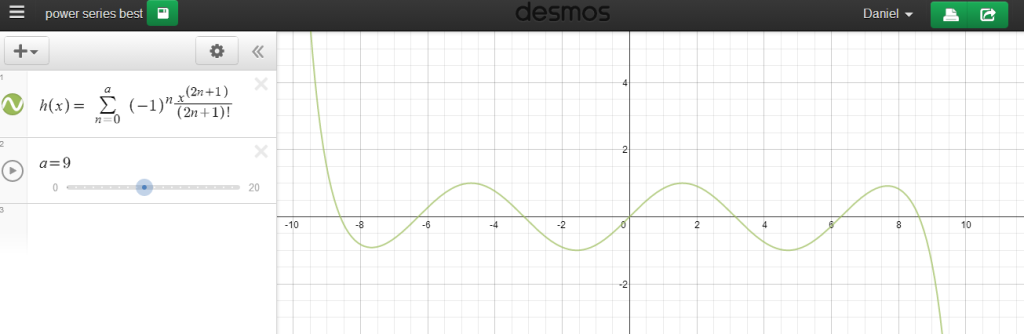Power Series work in AP Calculus BC.$\sum_{n=0}^{\infty}(-1)^{n}\frac{x^{2n+1}}{(2n+1)!}=\frac{x}{1!}-\frac{x^3}{3!}+\frac{x^5}{5!}-...$

Process: Since it’s a infinite series, look at partial sums to get an idea what this graph looks like.
So look at$y_1=\frac{x}{1!}$$y_2=\frac{x}{1!}-\frac{x^3}{3!}$$y_2=\frac{x}{1!}-\frac{x^3}{3!}+\frac{x^5}{5!}$

Perfect time to use technology.

## Texas Instruments Method

Go to y1. Enter in$y=x$.
Graph.
Wait 3-5 seconds.
Go to y1. Subtract a$\frac{x^3}{3!}$.
Graph.
Wait 3-5 seconds.
Go to y1. Add on a$\frac{x^5}{5!}$.
Graph.
Wait 3-5 seconds.
Go to y1. Subtract a$\frac{x^7}{7!}$.REALLY crappy resolution. Awful zoom system. Where’s the factorial sign? Hopefully you remember what the previous graphs looked like. Lots of waiting. Ugh.

## Desmos: version 1

Graph$y=x$
(NO WAIT STEP)
Subtract a$\frac{x^3}{3!}$.
(STILL NO WAIT STEP)
Add on a$\frac{x^5}{5!}$.
Subtract a$\frac{x^7}{7!}$.Great!

## Desmos: version 2

Students teaching teachers: Have one your students find this out for himself, and remark that they can enter in the entire series, but he’s having trouble finding the infinity sign. SLIDERS!